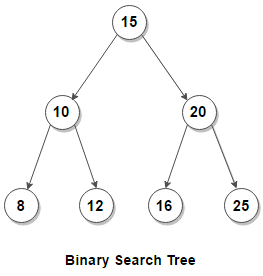# How To Build a Binary Search Tree from a Postorder Sequence

Given a distinct sequence of keys which represents postorder traversal of a binary search tree, construct the tree from the postorder sequence.

For example, below BST should be constructed for postorder traversal {8, 12, 10, 16, 25, 20, 15}For a given postorder sequence, we can easily build a BST by recursively repeating the following steps for all keys in it starting from the right.
1. Construct the root node of BST which would be the last key in the postorder sequence.

2. Find index i of the last key in the postorder sequence which is smaller than the root node.

3. Recur for right subtree with keys in the postorder sequence that appears after the i’th index (excluding last index).

4. Recur for left subtree with keys in the postorder sequence that appears before the i’th index (including i’th index).

To make context more clear, let’s consider the postorder traversal {8, 12, 10, 16, 25, 20, 15}
1. The last item in the postorder sequence 15 becomes the root node.

2. Since 10 is the last key in the postorder sequence which smaller than the root node, left subtree consists of keys {8, 12, 10} and right subtree consists of keys {16, 25, 20}.

3. To construct the complete binary search tree, recursively repeat the above steps for postorder sequence {8, 12, 10} and {16, 25, 20}.

This is demonstrated below in C –

Output:

Inorder Traversal of BST is : 8 10 12 15 16 20 25

The time complexity of above solution is O(n2) and takes O(n) extra space for the call stack. We can reduce the time complexity to O(n) by following a different approach that doesn’t involve searching for index which separates the keys of left and right subtree in postorder sequence.
We know that in a BST, each node has key which is greater than all keys present in its left subtree, and less than the keys present in the right subtree. The idea to pass the information regarding the valid range of keys for current root node and its children in the recursion itself.
We start by setting the range as [INT_MIN, INT_MAX] for the root node. This means that the root node and any of its children can have keys in the ranging between INT_MIN and INT_MAX. Like previous approach, we construct the root node of BST from the last item in the postorder sequence. Suppose the root node has value x, we recur for right subtree with range (x, INT_MAX) and recur for left subtree with range [INT_MIN, x). To construct the complete binary search tree, we recursively set the range for each recursive call and simply return if next element of postorder traversal is out of the valid range.
Here’s a C++ program that demonstrates it:

Output:

Inorder Traversal of BST is : 8 10 12 15 16 20 25